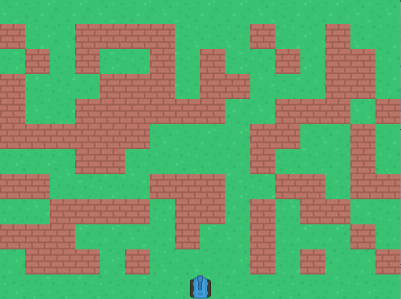# Random walls

To make the walls appear randomly, we'll first need to import the random module.

`import random`

Next, we'll choose a random number. The range is up to you, but I'm going with 0 to 100.

`random.randint(0, 100)`

...and we'll draw the wall only if the random number meets a certain condition, for example, if it is less than 50.

``````for x in range(16):
for y in range(10):
if random.randint(0, 100) < 50:
wall = Actor('wall')
wall.x = x * 50 + 25
wall.y = y * 50 + 25 + 50
walls.append(wall)
``````Since the wall pieces are drawn randomly, your screen may not look the same as mine.